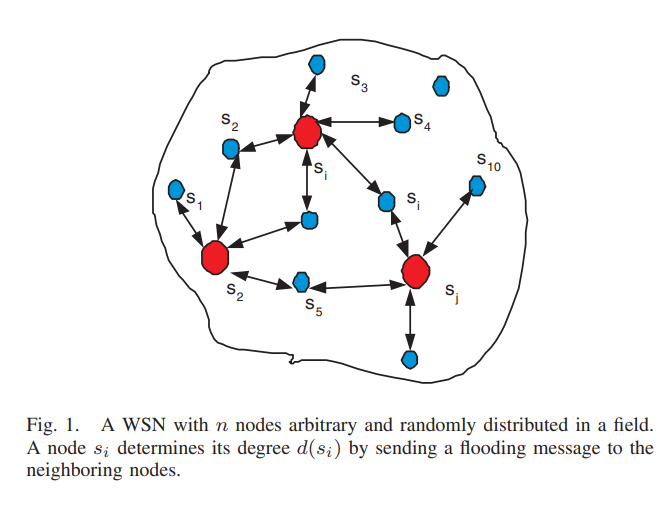Conference Paper

# Distributed flooding-based storage algorithms for large-scale wireless sensor networks

By
Aly S.A.
Youssef M.
Darwish H.S.
Zidan M.

In this paper we propose distributed storage algorithms for large-scale wireless sensor networks. Assume a wireless sensor network with n nodes that have limited power, memory, and bandwidth. Each node is capable of both sensing and storing data. Such sensor nodes might disappear from the network due to failures or battery depletion. Hence it is desired to design efficient schemes to collect data from these n nodes. We propose two distributed storage algorithms (DSA's) that utilize network flooding to solve this problem. In the first algorithm, DSA-I, we assume that the total number of sensors is known to each sensor in the network. We show that this algorithm is efficient in terms of the encoding and decoding operations. Furthermore, every node utilizes network flooding to disseminate its data throughout the network using a mixing time of approximately O(n). In the second algorithm, DSA-II, we assume that the total number of nodes is not known to every sensor; hence dissemination of the data does not depend on n. The encoding operations in this case take O(Cμ2), where μ is the mean degree of the network graph and C is a system parameter. We evaluate the performance of the proposed algorithms through analysis and simulation. We show that the performance of the proposed algorithms matches the derived theoretical results. ©2009 IEEE.